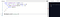Image credits to Ella Wei at Pexels

# Floyd’s Tortoise and Hare Algorithm — for beginners

I’ve been brushing up on my algorithms by focusing on Leetcode Medium problems — or trying to, at least. I came across the Find the Duplicate Number question and learned about the Floyd’s Tortoise and Hare solution. It was a bit challenging to grasp, but with the help of some very useful art and re-reading the code, I decided to share how and why this particular algorithm is so helpful (and time efficient!)

`Given an array of integers nums containing n + 1 where each integer is in the range [1, n] inclusive. There is only one repeated number in nums, return this repeated number.`

My first thought was to iterate through this array, and use the JavaScript method `indexOf`. `IndexOf` returns the index of the element if it can be found. I thought if I could iterate through the array and `indexOf` does not return -1, it would mean the number is not unique and therefore return nums[i].

Spoiler alert: I did say this was my first approach! This approach did not work, and that’s because it returned the value at the nums array at the zeroth index. Since nums at the initial index (which is 0) is 1, it is technically true that it is not equal to -1, and so this code would return the first value that this is true.Proof that my initial approach doesn’t work!

I took a second look at the constraints: we can’t modify the array and should be using constant O(1) time. Finally, I gave in and checked the solutions. To my surprise, I saw a number of solutions use something called Floyd’s Tortoise and Hare Algorithm.

Even after taking a look at their solution and watching a YouTube walkthrough, I didn’t understand how this approach was optimal. That’s when I came across Alisa Bajramovic’s blog post on Dev. I found it helpful, especially the diagram, but when I went back to my code, I was still confused.

`var findDuplicate = function(nums) {  let tort = nums  let hare = nums[tort]  while (tort !== hare){    tort = nums[tort]    hare = nums[nums[hare]]  }    tort = 0  while (tort !== hare){    tort = nums[tort]    hare = nums[hare]  }  return hare}`

We start by instantiating two variables, `tort` and `hare`. `Tort` is assigned the value of the nums array at index 0, and `hare` is assigned the value of nums at tort, which is one ahead, so at index 1. While the value of `tort` does not equal the value of `hare`, `tort` is the value of the next element (which is where hare was previously), and `hare` is the value of nums at the third element, or rather two indices ahead.

`Tort` is then the value of 0.

While `tort` is not equal to `hare`, `tort` is assigned the value of nums at the tort index, and then `hare` is assigned the value of nums at the `hare` index.

At the end of the while loop, you’ll return the variable `hare`.

Using this algorithm, we begin with `tort` assigned the value of 1, and `hare` to the value of 3. Since 1 !== 3, `tort` is now the value of 3, and `hare` has the updated value of 2 since it is at index 3. Again, these are not equal. You’ll continue to go through the array until `tort` reaches the end of the array — and since `hare` is the same value at `tort`, we return `hare`, or the value of 2.

This algorithm has a runtime of O(N), with the main factor that impacts runtime being the length of the array.

I’ve focused on building lately, and the focus on algorithms has been a great change of pace. These algorithms challenged me to consider different ways to achieve the solution — and it’s been even more challenging to find the optimal one! I’ve linked Alisa’s blog below, as I found the explanation and diagram helpful.

Full Stack Software Developer | aimeeliang.com

## More from Aimee Liang

Full Stack Software Developer | aimeeliang.com